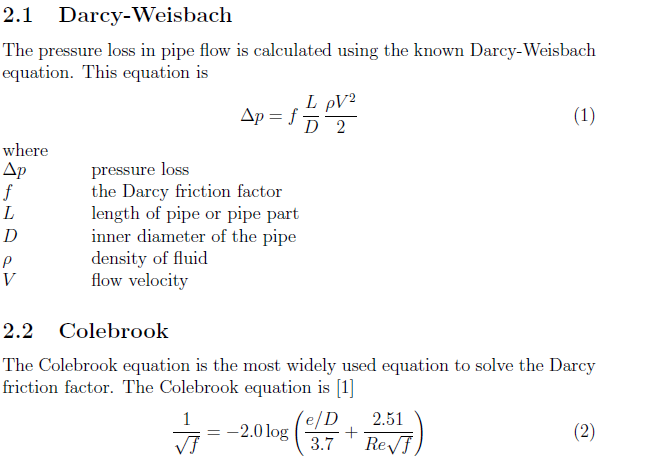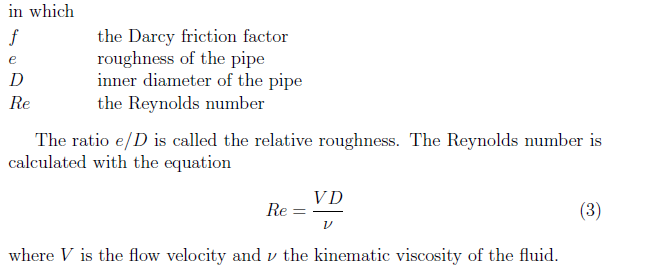# Friction coefficient "f" in pipes - Colebrook Darcy

Hi, good morning:

I am trying to obtain the friction coefficient of this following problem:

https://www.simscale.com/workbench/?pid=1746706367871332136&mi=spec%3A576b9d01-4829-4e13-9178-8a9c8442cbd4%2Cservice%3ASIMULATION%2Cstrategy%3A5

I know theorically is f=0.02781 but i would like to obtain it numerically by Simscale. However, i can’t find it on the postprocessing. How could I see it?

Besides this, I would like to see how much the entry length of the flow is at the beginnig of the pipe. Theorically, the value is 15.04 m considering turbulent flow.

Thanks for all!

Regards,

Andrea.

1 Like

You can calculate the Darcy friction factor using the following formula. You can find pressure loss by applying the area average result control to the inlet and outlet and calculate the difference in pressure. Also you can find the darcy friction factor from Colebrook equation if you know the roughness of the pipe you are simulating. I think, you cannot obtain friction factor directly from SimScale’s post processor2 Likes

I’m not aware of there being a way to find out the friction factor through the simulation but you can SET the wall roughness under custom boundary conditions.

See the following excellent tutorial/validation case

https://www.simscale.com/docs/content/validation/TurbulentPipeFlow/TurbulentPipeFlow.html

Also see this post about wall functions

2 Likes